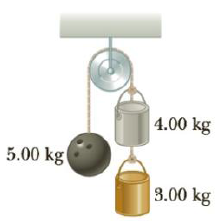Chapter 4, Problem 78AP

Chapter
Section
Textbook Problem

Three objects are connected by light strings as shown in Figure P4.78. The string connecting the 4.00-kg object and the 5.00-kg object passes over a light frictionless pulley. Determine (a) the acceleration of each object and (b) the tension in the two strings.Figure P4.78

(a)

To determine
The acceleration of the masses.

Explanation

Given Info: The masses are m1=5.00kg , m2=4.00kg and m3=3.00kg .

T1m1g=m1a (I)

T2T1+m2g=m2a (II)

m3gT2=m3a (III)

• T1 , T2 are the tension in the cords.
•  a is the acceleration of the masses.
• g is the acceleration due to gravity.

From Equations (I), (II) and (III),

a=g(m1+m2+m3m1+m2+m3) (IV)

Substitute 5

(b)

To determine
The tension on the two cords.

Still sussing out bartleby?

Check out a sample textbook solution.

See a sample solution

The Solution to Your Study Problems

Bartleby provides explanations to thousands of textbook problems written by our experts, many with advanced degrees!

Get Started

Today, an estimated 69 percent of the adults in the United States are overweight or obese.

Nutrition: Concepts and Controversies - Standalone book (MindTap Course List)

What are some characteristics of the adhesive used for Post-it Notes?

General Chemistry - Standalone book (MindTap Course List)

Calculate your protein RDA.

Understanding Nutrition (MindTap Course List)

What makes a tumor cell different from a normal cell?

Introduction to General, Organic and Biochemistry

Why can’t a white dwarf have a mass greater than 1.4 solar masses?

Horizons: Exploring the Universe (MindTap Course List)

What is physically exchanged during crossing over?

Human Heredity: Principles and Issues (MindTap Course List)